###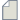Author Topic: problems about electron transport calculations  (Read 1583 times)

0 Members and 1 Guest are viewing this topic.

####heguangmeng

• New ATK user
•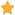• Posts: 5
• Country: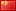• Reputation: 0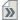##### problems about electron transport calculations
« on: April 27, 2018, 05:09 »
dear all
When we calculate a transmission of a device, the process shown in https://docs.quantumwise.com/tutorials/atk_transport_calculations/atk_transport_calculations.html is that "An ATK device calculation is performed in multiple steps. First, the electrode(s) and the central region are treated as (periodic) bulk systems, and the self-consistent electron density is calculated for each of them. The device calculation is then started, using the central region bulk electron density as an initial guess, and matching the electronic structure at the two electrode/central region boundaries to that of the electrodes. Finally, the self-consistent electronic structure of the central region is determined, and thereby of the full device. "
My questions are the method to  calculate the self-consistent electron density is  Hamiltonian matrices or other else? And the electrode will be calculated in the same method? Where can we set the intruct?

####Petr Khomyakov

• QuantumATK Staff
• Supreme ATK Wizard
•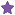• Posts: 1290
• Country:• Reputation: 25##### Re: problems about electron transport calculations
« Reply #1 on: April 27, 2018, 13:33 »
My questions are the method to  calculate the self-consistent electron density is  Hamiltonian matrices or other else? And the electrode will be calculated in the same method? Where can we set the intruct?